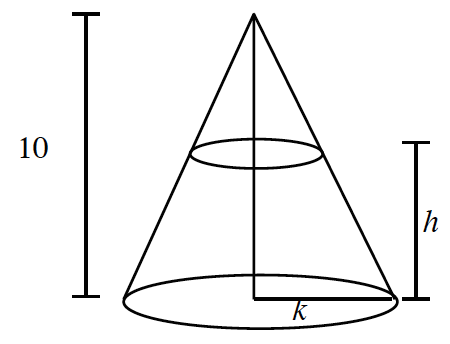### Home > PC > Chapter 5 > Lesson 5.2.4 > Problem5-88

5-88.

A cone has a height of $10$ inches and a radius of $k$ inches. If a plane cuts the cone $h$ inches above the base of the cone, in terms of $h$ and $k$ what is area of the circular cross section?1. Label the sides of the smaller triangle.
2. Then write a proportion between the sides of the smaller triangle and the larger triangle.
3. Solve for $x$.